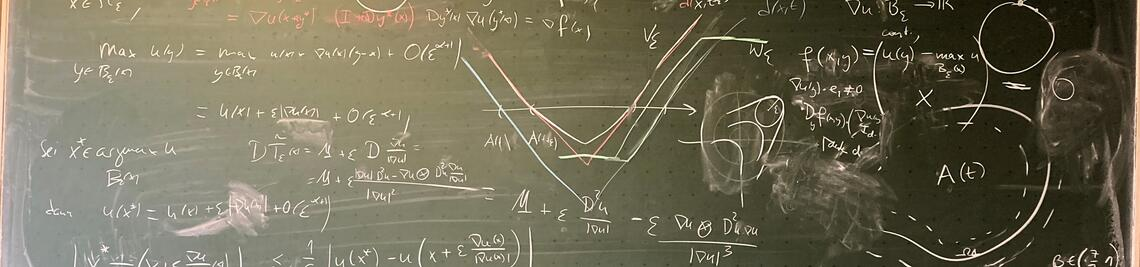•# Research areas

### Adversarial robustness of machine learning

We are investigating mathematical models for describing and analyzing adversarial attacks on artificial intelligence. This topic has strong connections to regularization techniques from the field of inverse problems, as well as to partial differential equations and geometric flows.

### Partial differential equations on graphs

Graphs are discrete structures consisting of a set of nodes connected by weighted edges. They can be used to model and analyze problems related to semi-supervised learning. We are working on questions that relate these discrete models to continuous ones, typically involving partial differential equations.

### Optimization

We are working on various topics in the field of optimization that are relevant to machine learning. This includes sparse optimization to train neural networks with few parameters. Additionally, we are exploring optimization with swarm methods. These approaches are particularly interesting when the function to be optimized is only available as a black box, is non-differentiable or costly to differentiate, or exhibits highly non-convex behavior.